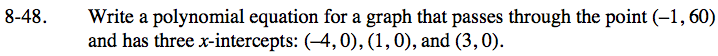### Home > INT3 > Chapter Ch8 > Lesson 8.1.3 > Problem8-48

8-48.

Write a polynomial equation for a graph that passes through the point (–1, 60) and has three x-intercepts: (–4, 0), (1, 0), and (3, 0). Homework Help ✎Start by writing an equation in factored form.
p(x) = a(x + 4)(x − 1)(x − 3)

Find the stretch factor by substituting the point.

$(60) = a((-1) + 4)((-1)-1)((-1)-3)$

$y = \frac{5}{2}(x + 4)(x - 1)(x - 3)$